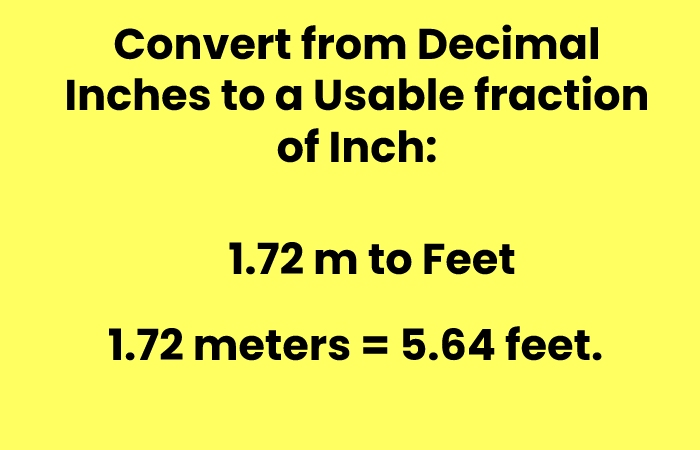## 1.72 m to Feet

1.72 meters to feet shows you how many feet are equal to 1.72 meters as well as in other units such as miles, inches, yards, centimeters, and kilometers.

## What are 1.72 m to Feet?

1.72 meters equals 5 feet and 8 inches or 5.64 feet. One meter equals roughly 3.28 feet, and there are 12 inches in a foot.

 Other Conversions Meters: 1.72 Feet: 5.64304 inches: 67.71657 Feet & Inches: 5′ 7.72″ Miles 0.00107 Yards: 1.88101 Kilometers: 0.00172 Centimeters: 172.00000

## Formula

Ft = meters × 3.28084According to the ‘meters to feet’ conversion formula, if you want to convert 1.72 (one point seven two) Meters to Feet, you have to multiply 1.72 by 3.28084.

Complete solution:1.72 m × 3.28084=5.64′If you want to convert 1.72 M to both Feet and Inches parts, then you first have to calculate the whole number part for Feet by rounding 1.72 × 3.28084 fractions down.

• And then convert the remainder of the division to Inches by multiplying by 12 (according to the Feet to Inches conversion formula).

Complete solutions: ( 1.72 meters × 3.28084 )=5′get the Inches Part((1.72 × 3.28084) – 5′) * 12=(5.643 – 5′) * 12=0.643 * 12=7.72″so the full record will look like5′7.72″.

## Step 1

### Convert from meters to feet:

• 1 meter = 3.28 x feet, so,
• 1.72 x 1 meter = 1.72 x 3.28 feet, or
• 1.72 meters = 5.64 feet.

## Step 2

### Convert the decimal feet to inches:

• An answer like “5.64 feet” might not mean much to you because you may want to express the decimal part, which is in feet, in inches once it is a smaller unit.
• So, take everything after the decimal point (0.64), then multiply that by 12 to turn it into inches. It works because one foot = 12 inches. Then,
• 5.64 feet = 5 feet + 0.64 foot. Once 0.64 foot x 12 = 7.68 inches or 5.64 feet = 5 feet and 7.68 inches. It is equivalent to 1.72 meters.

## Step 3

### Convert from decimal inches to a usable fraction of inch:The previous step gave you the answer in decimal inches (7.68), but how to measure it with a ruler or tape? See below a procedure, which can also be made using a calculator, to convert the decimal inches to the nearest usable fraction:

Subtract 7, the number of whole inches, from 7.68:

• 7.68 – 7 = 0.68. It is the fractional part of the inch value.

Multiply 0.68 times 16 (it could be 8, 16, 32, 64, depending on the exactness you want) to get the number of 16th inches:

• 0.68 x 16 = 10.88. It is the number of 16ths of an inch and the fraction’s numerator, which may be still reduced.

Round the result to the nearest integer:

• round(10.88) = 11
• Finally, 1.72 meters = 5 feet and 11/16 of an inch.

Hence: 1.72 m = 5’7 11/16″

## Meters to Feet Table

 Meters Feet 1.00 m 3.281 ft 1.01 m 3.314 ft 1.02 m 3.346 ft 1.03 m 3.379 ft 1.04 m 3.412 ft 1.05 m 3.445 ft 1.06 m 3.478 ft 1.07 m 3.510 ft 1.08 m 3.543 ft 1.09 m 3.576 ft 1.10 m 3.609 ft 1.11 m 3.642 ft 1.12 m 3.675 ft 1.13 m 3.707 ft 1.14 m 3.740 ft 1.15 m 3.773 ft 1.16 m 3.806 ft